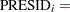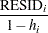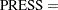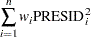Predicted and Residual Values

After the model has been fit, predicted and residual values are usually calculated, graphed, and output. The predicted values are calculated from the estimated regression equation; the raw residuals are calculated as the observed minus the predicted value. Often other forms of residuals are used for model diagnostics, such as studentized or cumulative residuals. Some procedures can calculate standard errors of residuals, predicted mean values, and individual predicted values.

Consider theth observation where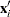is the row of regressors,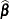is the vector of parameter estimates, and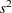is the estimate of the residual variance (the mean squared error). The leverage value of theth observation is defined as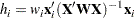whereis the design matrix for the observed data,is an arbitrary regressor vector (possibly but not necessarily a row of),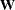is a diagonal matrix with the observed weights on the diagonal, and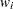is the weight corresponding to.

Then the predicted mean and the standard error of the predicted mean are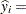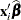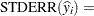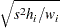The standard error of the individual (future) predicted value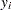is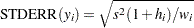If the predictor vector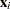corresponds to an observation in the analysis data, then the raw residual for that observation and the standard error of the raw residual are defined as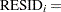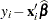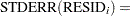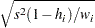The studentized residual is the ratio of the raw residual and its estimated standard error. Symbolically,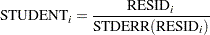There are two kinds of intervals involving predicted values that are associated with a measure of confidence: the confidence interval for the mean value of the response and the prediction (or forecasting) interval for an individual observation. As discussed in the section Mean Squared Error in Chapter 3, Introduction to Statistical Modeling with SAS/STAT Software, both intervals are based on the mean squared error of predicting a target based on the result of the model fit. The difference in the expressions for the confidence interval and the prediction interval comes about because the target of estimation is a constant in the case of the confidence interval (the mean of an observation) and the target is a random variable in the case of the prediction interval (a new observation).

For example, you can construct a confidence interval for theth observation that contains the true mean value of the response with probability
. The upper and lower limits of the confidence interval for the mean value are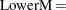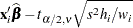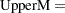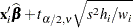where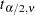is the tabulatedquantile with degrees of freedom equal to the degrees of freedom for the mean squared error,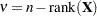.

The limits for the prediction interval for an individual response are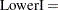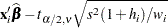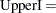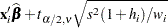Influential observations are those that, according to various criteria, appear to have a large influence on the analysis. One measure of influence, Cook’s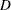, measures the change to the estimates that results from deleting an observation: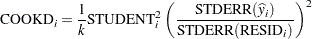whereis the number of parameters in the model (including the intercept). For more information, see Cook (1977, 1979).

The predicted residual for observationis defined as the residual for theth observation that results from dropping theth observation from the parameter estimates. The sum of squares of predicted residual errors is called the PRESS statistic: